﻿ 飞机起降滑行过程中的非线性振动及其时滞反馈控制研究 Study on Nonlinear Vibration and Delayed Feedback Control of Aircraft in Take-Off and Sliding

Journal of Aerospace Science and Technology
Vol.05 No.03(2017), Article ID:22195,12 pages
10.12677/JAST.2017.53018

Study on Nonlinear Vibration and Delayed Feedback Control of Aircraft in Take-Off and Sliding

Cheng Dong, Dunke Song, Yanying Zhao*

School of Aircraft Engineer, Nanchang Hangkong University, Nanchang Jiangxi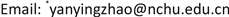Received: Sep. 8th, 2017; accepted: Sep. 22nd, 2017; published: Sep. 28th, 2017ABSTRACT

In the present paper, the vertical nonlinear vibration characteristics of landing gear system are investigated when the aircraft is taxiing. The delayed feedback control is employed to control and suppress the vibration of the aircraft body. The differential equations of a two degree of freedom nonlinear vibrating system is obtained and approximate analytical solution is achieved by the method of decouping and multiple scales. The dynamical behavior of vibrating system is investigated when the external frequency approaches the first order modal frequency and 1:5 internal resonance occurs simultaneously. The focus is to analyze the effect of gain and time delay on the vibration of the vibrating system. The response curve of primary system and time delay is obtained and the stability of the system is investigated. The results show that the vibration of the aircraft body is suppressed about 48% when the controlling parameters are chosen appropriately. However, the vibration of the aircraft body may lose its stability and destroyed when the controlling parameters are chosen unreasonably. The results of the present paper may improve the vibrating theory of aircraft landing gear system and provide valuable reference for engineering designers.

Keywords:Landing Gear, Vibration Reduction, Nonlinear, Time-Delay Feedback Control1. 引言

2. 起落架系统运动微分方程的建立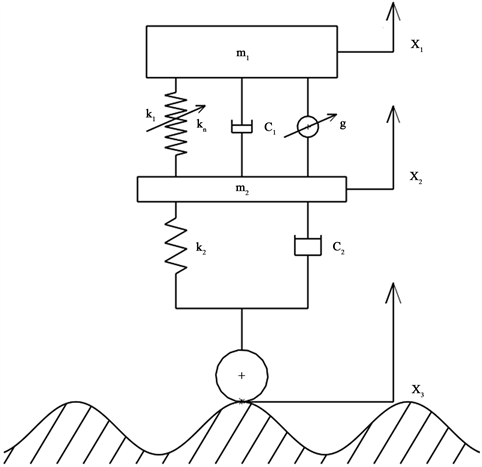Figure 1. Schematic diagram of landing gear system model

$\left\{\begin{array}{l}{m}_{1}{\stackrel{¨}{x}}_{1}={k}_{1}\left({x}_{2}-{x}_{1}\right)+{k}_{n}{\left({x}_{2}-{x}_{1}\right)}^{5}+{c}_{1}\left({\stackrel{˙}{x}}_{2}-{\stackrel{˙}{x}}_{1}\right)-g{\stackrel{˙}{x}}_{1}\left(t-\tau \right)\\ {m}_{2}{\stackrel{¨}{x}}_{2}={k}_{2}\left({x}_{3}-{x}_{2}\right)+{c}_{2}\left({\stackrel{˙}{x}}_{3}-{\stackrel{˙}{x}}_{2}\right)-{k}_{1}\left({x}_{2}-{x}_{1}\right)-{k}_{n}{\left({x}_{2}-{x}_{1}\right)}^{5}-{c}_{1}\left({\stackrel{˙}{x}}_{2}-{\stackrel{˙}{x}}_{1}\right)+g{\stackrel{˙}{x}}_{1}\left(t-\tau \right)\end{array}$

${x}_{3}=F\mathrm{sin}\left(\omega t+{\varphi }_{1}\right)$${k}_{2}{x}_{3}+{c}_{2}{\stackrel{˙}{x}}_{3}={k}_{2}F\mathrm{sin}\left(\omega t+{\varphi }_{1}\right)+{c}_{2}\omega F\mathrm{cos}\left(\omega t+{\varphi }_{1}\right)=f\mathrm{cos}\left(\omega t+{\varphi }_{2}\right)$ ，为了计算方便，可通过构造合适的 ${\varphi }_{1}$ 使得 ${\varphi }_{2}=0$ ，故矩阵可写成如下形式：

$\begin{array}{l}\left[\begin{array}{cc}{m}_{1}& 0\\ 0& {m}_{2}\end{array}\right]\left[\begin{array}{c}{\stackrel{¨}{x}}_{1}\\ {\stackrel{¨}{x}}_{2}\end{array}\right]+\left[\begin{array}{cc}{c}_{1}& -{c}_{1}\\ -{c}_{1}& {c}_{1}+{c}_{2}\end{array}\right]\left[\begin{array}{c}{\stackrel{˙}{x}}_{1}\\ {\stackrel{˙}{x}}_{2}\end{array}\right]+\left[\begin{array}{cc}{k}_{1}& -{k}_{1}\\ -{k}_{1}& {k}_{1}+{k}_{2}\end{array}\right]\left[\begin{array}{c}{x}_{1}\\ {x}_{2}\end{array}\right]\\ +\left[\begin{array}{c}{k}_{n}{\left({x}_{1}-{x}_{2}\right)}^{5}+g{\stackrel{˙}{x}}_{2}\right)\end{array}\right]\\ \text{\hspace{0.17em}}+\frac{1}{2}\left({r}_{1}-1\right){\omega }_{1}g\left[\mathrm{sin}\left({\omega }_{1}\tau \right){s}_{1}+\mathrm{cos}\left({\omega }_{1}\tau \right){d}_{1}\right]+\frac{1}{2}{r}_{1}f\right\}\end{array}$ (4b)

$\begin{array}{c}{{s}^{\prime }}_{2}={b}^{\prime }\mathrm{cos}\left({\varphi }_{1}-5{\varphi }_{2}\right)-b\left({{\varphi }^{\prime }}_{1}-5{{\varphi }^{\prime }}_{2}\right)\mathrm{sin}\left({\varphi }_{1}-5{\varphi }_{2}\right)\\ =\frac{1}{\left({m}_{1}+{r}_{2}^{2}{m}_{2}\right){\omega }_{2}}\left\{-\left[{c}_{2}{r}_{2}^{2}+{c}_{1}{\left({r}_{2}-1\right)}^{2}\right]\frac{{s}_{2}{\omega }_{2}}{2}-\left({m}_{1}+{r}_{2}^{2}{m}_{2}\right){\omega }_{2}{d}_{2}\left({\sigma }_{2}-5{\sigma }_{1}\right)\\ \text{\hspace{0.17em}}\text{\hspace{0.17em}}-\frac{15{\left({s}_{1}^{2}+{d}_{1}^{2}\right)}^{2}}{16}{\left({r}_{1}-1\right)}^{4}{\left({r}_{2}-1\right)}^{2}{k}_{n}{d}_{2}-\frac{15\left({s}_{1}^{2}+{d}_{1}^{2}\right)\left({s}_{2}^{2}+{d}_{2}^{2}\right)}{8}{\left({r}_{1}-1\right)}^{2}{\left({r}_{2}-1\right)}^{4}{k}_{n}{d}_{2}\\ \text{\hspace{0.17em}}\text{\hspace{0.17em}}-\frac{5{\left({s}_{2}^{2}+{d}_{2}^{2}\right)}^{2}}{16}\left({r}_{2}-1\right){k}_{n}{d}_{2}-\frac{1}{32}{\left({r}_{1}-1\right)}^{5}\left({r}_{2}-1\right){k}_{n}\left[4{d}_{1}^{3}{s}_{1}^{2}-4{d}_{1}^{5}-8{d}_{1}{s}_{1}^{4}+3{\left({s}_{1}^{2}+{d}_{1}^{2}\right)}^{2}{d}_{1}\right]\\ \text{\hspace{0.17em}}\text{\hspace{0.17em}}+\frac{1}{2}\left({r}_{2}-1\right){\omega }_{2}g\left[\mathrm{sin}\left({\omega }_{2}\tau \right){d}_{2}+\mathrm{cos}\left({\omega }_{2}\tau \right){s}_{2}\right]\right\}\end{array}$ (4c)

$\begin{array}{c}{{d}^{\prime }}_{2}={b}^{\prime }\mathrm{sin}\left({\varphi }_{1}-5{\varphi }_{2}\right)+b\left({{\varphi }^{\prime }}_{1}-5{{\varphi }^{\prime }}_{2}\right)\mathrm{cos}\left({\varphi }_{1}-5{\varphi }_{2}\right)\\ =\frac{1}{\left({m}_{1}+{r}_{2}^{2}{m}_{2}\right){\omega }_{2}}\left\{-\left[{c}_{2}{r}_{2}^{2}+{c}_{1}{\left({r}_{2}-1\right)}^{2}\right]\frac{{d}_{2}{\omega }_{2}}{2}+\left({m}_{1}+{r}_{2}^{2}{m}_{2}\right){\omega }_{2}\left({\sigma }_{2}-5{\sigma }_{1}\right){s}_{2}\\ \text{\hspace{0.17em}}\text{\hspace{0.17em}}+\frac{15{\left({s}_{1}^{2}+{d}_{1}^{2}\right)}^{2}}{16}{\left({r}_{1}-1\right)}^{4}{\left({r}_{2}-1\right)}^{2}{k}_{n}{s}_{2}+\frac{15\left({s}_{1}^{2}+{d}_{1}^{2}\right)\left({s}_{2}^{2}+{d}_{2}^{2}\right)}{8}{\left({r}_{1}-1\right)}^{2}{\left({r}_{2}-1\right)}^{4}{k}_{n}{s}_{2}\\ \text{\hspace{0.17em}}\text{\hspace{0.17em}}\text{\hspace{0.17em}}+\frac{5{\left({s}_{2}^{2}+{d}_{2}^{2}\right)}^{2}}{16}\left({r}_{2}-1\right){k}_{n}{s}_{2}+\frac{1}{32}{\left({r}_{1}-1\right)}^{5}\left({r}_{2}-1\right){k}_{n}\left[4{s}_{1}^{5}-4{s}_{1}^{3}{d}_{1}^{2}+8{d}_{1}^{4}{s}_{1}-3{\left({s}_{1}^{2}+{d}_{1}^{2}\right)}^{2}{s}_{1}\right]\\ \text{\hspace{0.17em}}\text{\hspace{0.17em}}-\frac{1}{2}\left({r}_{2}-1\right){\omega }_{2}g\left[\mathrm{sin}\left({\omega }_{2}\tau \right){s}_{2}-\mathrm{cos}\left({\omega }_{2}\tau \right){d}_{2}\right]\right\}\end{array}$ (4d)

$\epsilon$ 很小时，主系统和副系统的位移可以表示成如下的形式：

${x}_{1}={q}_{1}+{q}_{2}={q}_{11}+{q}_{21}+\epsilon \left({q}_{12}+{q}_{22}\right)+\cdot \cdot \cdot \approx a\mathrm{cos}\left[{\omega }_{1}{T}_{0}+{\theta }_{1}\left({T}_{1}\right)\right]+b\mathrm{cos}\left[{\omega }_{2}{T}_{0}+{\theta }_{2}\left({T}_{1}\right)\right]$ (5a)

#Math_104#(5b)

${\mu }_{1}>0,\text{\hspace{0.17em}}{\mu }_{1}{\mu }_{2}-{\mu }_{3}>0,\text{\hspace{0.17em}}{\mu }_{3}\left({\mu }_{1}{\mu }_{2}-{\mu }_{3}\right)-{\mu }_{1}^{2}{\mu }_{4}>0,\text{\hspace{0.17em}}{\mu }_{4}>0$ (6)

5. 非线性系统时滞反馈的控制研究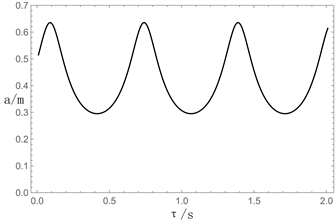Figure 2. $\omega ={\omega }_{1}-\epsilon {\sigma }_{1}$Figure 3. $\omega ={\omega }_{1}-\epsilon {\sigma }_{1}$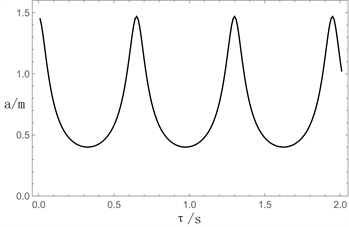Figure 4. $\omega ={\omega }_{1}$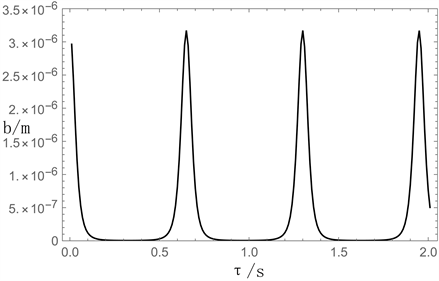Figure 5. $\omega ={\omega }_{1}$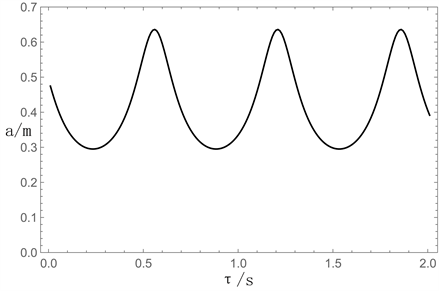Figure 6. $\omega ={\omega }_{1}+\epsilon {\sigma }_{1}$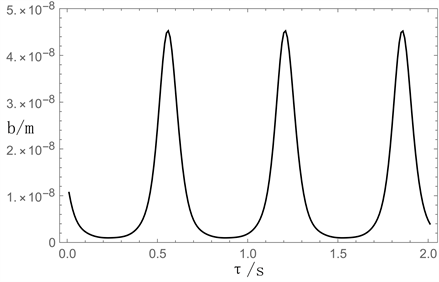Figure 7.Figure 8. $g=-8100\text{\hspace{0.17em}}\text{N}\cdot \text{s}/\text{m}$Figure 9. $g>-8100\text{\hspace{0.17em}}\text{N}\cdot \text{s}/\text{m}$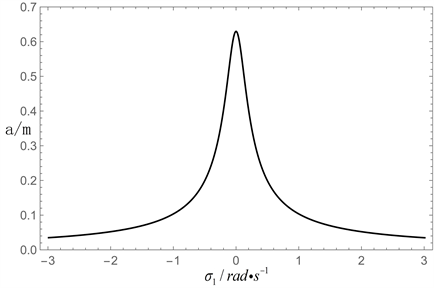Figure 10. $g=0\text{\hspace{0.17em}}\text{N}\cdot \text{s}/\text{m}$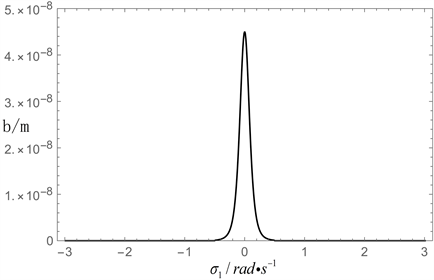Figure 11. $g=0\text{\hspace{0.17em}}\text{N}\cdot \text{s}/\text{m}$

6. 结论

1) 当反馈增益系数为某一定值时，在稳定范围内存在某些时滞量区间，时滞反馈控制可以对机体进行减振。并且存在一个“最大减振点”的时滞量，可以将机体的振动减到这一反馈增益系数下的最小。

2) 不同的反馈增益系数，其“最大减振点”对应机体的振幅也不同。当反馈增益系数在系统稳定的范围内改变时，可以找到一个最佳的反馈增益系数和对应的最佳时滞量，将机体的振动减到最小。

3) 在最佳时滞项下，与无时滞项的非线性被动系统相比，飞机机体的减振幅度约达48%，这说明时滞反馈控制对机体减振的有效性。

4) 本文中时滞项中的反馈增益系数和时滞量都是定值，不能随时间和外激励的情况而变化，而且当反馈增益系数和时滞量不断增大时，系统会越来越不稳定。同时，在系统稳定的区域内也存在某些区间会使机体振动变得更加剧烈。所以，时滞反馈控制具有一定的局限性。

5) 本文的参数是基于小参数法思想，得到的解只是近似的解析解，故计算结果与实际结果会存在一定的误差。

Study on Nonlinear Vibration and Delayed Feedback Control of Aircraft in Take-Off and Sliding[J]. 国际航空航天科学, 2017, 05(03): 163-174. http://dx.doi.org/10.12677/JAST.2017.53018

1. 1. 贾玉红, 郭可谦. 飞机起落架的发展[C]. 第一届中日机械技术史国际学术会议. 北京: 机械工业出版社, 1998.

2. 2. 聂宏. 起落架缓冲性能分析与设计及其寿命计算方法[D]: [博士学位论文]. 南京: 南京航空航天大学, 1990.

3. 3. 马魁江.飞机起落架结构耐久性设计[D]: [博士学位论文]. 南京: 南京航空航天大学, 2008.

4. 4. 随福成, 王成波. 飞机起落架延长使用寿命研究的新途径[J]. 沈阳飞机设计研究所, 2008, 62(8): 336-339.

5. 5. Olgac, N., McFarland, D.M. and Holm-Hansen, B.T. (1992) Position Feedback-Induced Resonance: The Delayed Resonator. Winter Annual Meeting, 38, 113-119.

6. 6. Renzulli, M.E., Roy, R.G. and Olgac, N. (1999) Robust Control of Delayed Resonator Virbration Absorber. IEEE Transactions on Control Systems Technology, 7, 683-691. https://doi.org/10.1109/87.799669

7. 7. Olgac, N., Hosek, M., et al. (1997) Active Vibration Absorption Using Delayed Resonator With Relative Position Measurement. Journal of Vibration & Acoustics, 119, 131-136. https://doi.org/10.1115/1.2889680

8. 8. Filipović, D. and Olgac, N. (2002) Delayed Resonator with Speed Feedback-Design and Performance. Mechatronics, 12, 393-413. https://doi.org/10.1016/S0957-4158(00)00065-9

9. 9. Shin, Y.H., et al. (2010) Three Degrees of Freedom Active Control Of Pneumatic Vibration Isolation Table by Pneumatic and Time Delay Control Technique. Journal of Vibration and Acoustics, 132, Article ID: 051013. https://doi.org/10.1115/1.4001509

10. 10. 薛华鑫. 飞机滑行状态下振动频率响应分析[D]: [硕士学位论文]. 天津: 中国民航大学, 2014.

11. NOTES



*通讯作者。